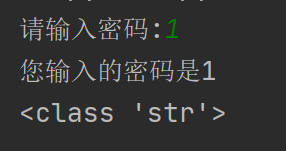﻿ python输入、数据类型转换及运算符方式_python_脚本之家
python# python输入、数据类型转换及运算符方式

## 一、输入

input("提示内容")

（1）当程序执行到input，等待用户输入，输入完成后才继续往下执行

（2）input接收用户输入后，一般存储到变量，方便使用

（3）input会把接收到的任意用户输入的数据都当作字符串处理## 二、常用转换数据类型的函数

int(x)将x转化为一个整数
float(x)将x转化为一个浮点数
str(x)将对象x转化为字符串
eval(str)用来计算在字符串中的有效python表达式，并返回一个对象
tuple(s)将序列s转化为一个元组
list(s)将序列s转化为一个列表

a = "1.1"，用eval函数处理a字符串，它会转换成浮点型

input（）用户输入内容默认为字符串型，转换为整型## 三、运算符

• 算术运算符
• 赋值运算符
• 复合赋值运算符
• 比较运算符
• 逻辑运算符

### 1.算术运算符

 运算符 描述 实例 + 加 1+1=2 - 减 1-1=0 * 乘 2*2=4 / 除 10/2=5 // 整除 9//4=2 % 取余 9%4=1 ** 指数 2**3=8，即2*2*2 () 小括号 小括号用来提高运算优先级

### 2.赋值运算符

（1）单个变量赋值

`num = 1`

（2）多个变量赋值

```a = b = 1
a, b, c = 1, 1.1, 'python'      （a，b，c会对应赋值为1 1.1 python）```

### 3.复合赋值运算符

 运算符 描述 实例 += 加法赋值运算符 a+=b等价于a=a+b -= 减法赋值运算符 a-=b等价于a=a-b *= 乘法赋值运算符 a*=b等价于a=a*b /= 除法赋值运算符 a/=b等价于a=a/b //= 整除赋值运算符 a//=b等价于a=a//b %= 取余赋值运算符 a%=b等价于a=a%b **= 幂赋值运算符 a**=b等价于a=a**b
```a=10
a *= 1+2```### 4.比较运算符

 运算符 描述 == 等于 != 不等于 > 大于 < 小于 >= 大于等于 <= 小于等于

### 5.逻辑运算符

 运算符 描述 and x and y,当x，y两个条件都为真时，返回True，否则返回False or x or y，当x，y两个条件都为假时，返回False，否则返回True not not x，x为真则返回假，x为假则返回真

（1）and运算符，只要有一个值为0，则结果为0，否则结果为最后一个非0数字（2）or运算符，两个值都为0时，结果为0，否则结果为第一个非0数字